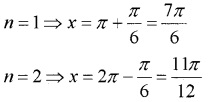# Plus One Maths Chapter Wise Questions and Answers Chapter 3 Trigonometric Functions

Students can Download Chapter 3 Trigonometric Functions Questions and Answers, Plus One Maths Chapter Wise Questions and Answers helps you to revise the complete Kerala State Syllabus and score more marks in your examinations.

## Kerala Plus One Maths Chapter Wise Questions and Answers Chapter 3 Trigonometric Functions

### Plus One Maths Trigonometric Functions Three Mark Questions and Answers

Question 1.
Prove the following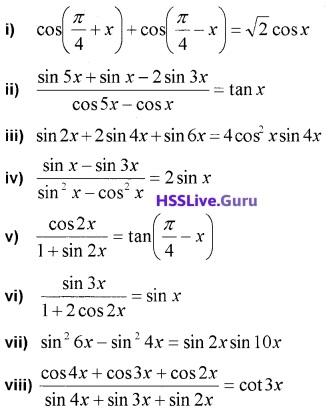i) LHSii) LHS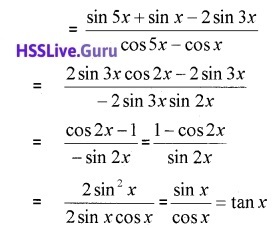iii) LHS = sin 2x + 2 sin 4x + sin 6x
= 2 sin 4xcos2x + 2sin 4x
= 2 sin 4x(cos2x + 1) = 4 cos2 x sin 4x

iv) LHS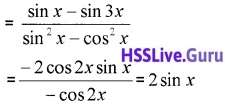v) LHSvi) LHSvii) LHS = sin2 6x – sin2 4x= 2 sin 10x sin(-2x)
= 2 sin 10x sin2x

viii) LHSQuestion 2.
Find the general solution of the following equations.

1. cos4x = cos2x
2. sin 2x +cosx = 0
3. cos3x + cosx – cos2x = 0

1. Given; cos 4x = cos 2x
⇒ cos4x – cos 2x = 0
⇒ -2 sin 3x sin x = 0
General solution is
⇒ sin3x = 0; ⇒ 3x = nπ ⇒ x = $$\frac{n \pi}{3}$$, ∈ Z
Again we have;
⇒ sinx = 0; ⇒ x = nπ; n ∈ Z

2. Given; sin 2x + cosx = 0
⇒ 2sin xcosx + cosx = 0
⇒ cosx(2sin x + 1) = 0
General solution is
⇒ cosx = 0 ⇒ x = (2n + 1) $$\frac{\pi}{2}$$, n ∈ Z
Again we have; 2sin x + 1 = 0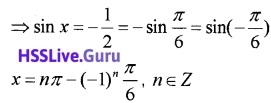3. Given; cos3x +cosx – cos2x = 0
⇒ 2 cos2x cosx – cos2x = 0
⇒ cos2x(2cosx – 1) = 0
General solution isAgain we have; 2cosx -1 = 0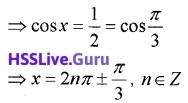Question 3.
In Triangle ABC, if a = 25, b = 52 and c = 63, find cos A and sin A.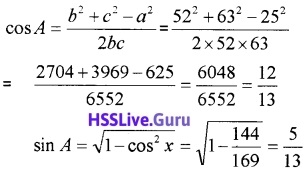Question 4.
For any ΔABC, prove that a(b cosC – c cosB) = b2 – c2
LHS = ab cos C – ac cos B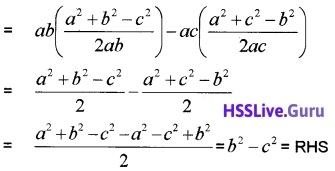Question 5.
For any ΔABC, prove that, $$\frac{\sin (B-C)}{\sin (B+C)}=\frac{b^{2}-c^{2}}{a^{2}}$$.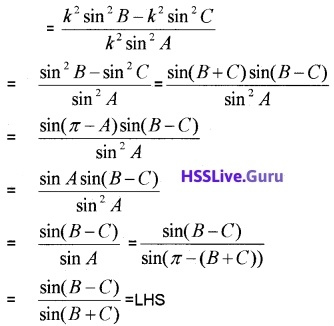Question 6.

1. Convert $$\frac{2 \pi}{3}$$ radian measure into degree measure. (1)
2. Prove that $$\frac{\sin 5 x+\sin 3 x}{\cos 5 x+\cos 3 x}=\tan 4 x$$ (2)

1. $$\frac{2 \pi}{3}=\frac{2 \pi}{3} \times \frac{180}{\pi}=120^{\circ}$$

2. LHS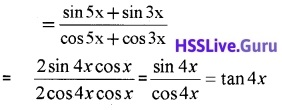### Plus One Maths Trigonometric Functions Four Mark Questions and Answers

Question 1.
For any ΔABC, prove that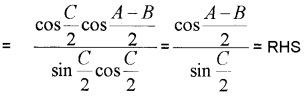Question 2.
For any ΔABC, prove that $$\sin \frac{B-C}{2}=\frac{b-c}{a} \cos \frac{A}{2}$$.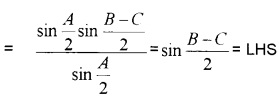Question 3.
(i) Which of the following is not possible. (1)
(a) sin x = $$\frac{1}{2}$$
(b) cos x = $$\frac{2}{3}$$
(c) cosec x = $$\frac{1}{3}$$
(d) tan x = 8
(ii) Find the value of sin 15°. (2)
(iii) Hence write the value of cos 75° (1)
(i) (c) cosec x = $$\frac{1}{3}$$

(ii) sin 15° = sin(45° – 30°)
= sin45°cos30°- cos45°sin30°(iii) sin 15° = sin(90° – 75°) = cos 75°

### Plus One Maths Trigonometric Functions Six Mark Questions and Answers

Question 1.
The angle of elevation of the top point P of the vertical tower PQ of height h from a point A is 45° and from a point B, the angle of elevation is 60°, where B is a point at a distance d from the point A measured along the line AB which makes angle 30° with AQ. Prove that d = h($$\sqrt{3}$$ – 1).From the figure we have ∠PAQ = 45°, ∠BAQ = 30°and ∠PBH = 60°
in right ∆AQP
Clearly ∠APQ = 45°, ∠BPH = 30° , giving ∠APB = 15° ⇒ ∠PAB = 15°
In ∆APQ ,PQ = AQ = h
AP2 = h2 + h2 = 2h2 ⇒ AP = $$\sqrt{2}$$h
From ∆ABP,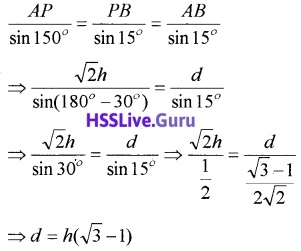Question 2.
A tree stands vertically on a hill side which makes an angle of 15° with the horizontal. From a point on the ground 35m down the hill from the base of the tree, the angle of elevation of the top of the tree is 60°. Find the height of the tree.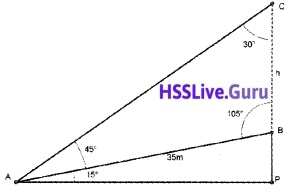Let BC represent the tree, A be the point 35m down the hill from the base of the tree and h be the height of the tree.
Clearly in ∆ABC
∠BAC = 60°- 15° =45°;
∠ACB = 30°; ∠ABC = 105°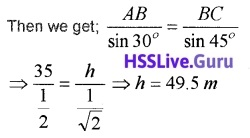Question 3.
(i) If sin x = cos x, x ∈ [0, π] then is
(a) 0
(b) $$\frac{\pi}{4}$$
(c) $$\frac{\pi}{3}$$
(d) π
(ii) Write the following in ascending order of tits values, sin 100°, sin 0°, sin 50°, sin 200°
(iii) Solve: sin2x – sin4x + sin6x = 0
(i) (b) $$\frac{\pi}{4}$$

(ii) sin 100° = sin(l 80 – 80) = sin 80°
sin 200° = sin(l 80° + 20°) = -sin 20°
The ascending order is
sin 200°, sin 0°, sin 50°, sin 100°

(iii) sin2x + sin6x – sin4x = 0
⇒ 2sin 4x cos2x – sin 4x = 0
⇒ sin 4x(2 cos 2x – 1) = 0
⇒ sin4x = 0 or (2cos2x – 1) = 0
⇒ 4x = nπ or cos2x = $$\frac{1}{2}$$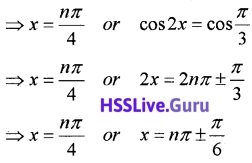### Plus One Maths Trigonometric Functions Practice Problems Questions and Answers

Question 1.
Convert the following degree measure into radian measure.
i)  45°
ii) 25°
iii) 240°
iv) 40°20′
v) -47°30′Question 2.
Convert the following radian measure into degree measure,
i)   6
ii) -4
iii) $$\frac{5 \pi}{3}$$
iv) $$\frac{7 \pi}{6}$$
v) $$\frac{11}{16}$$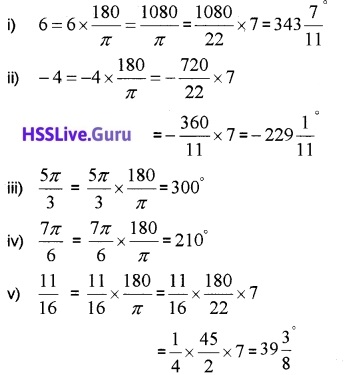Question 3.
The minute hand of a watch is 1.5 cm long. How far does its tip move in 40 minutes? (Use π = 3.14)
60 minutes = 360 degrees.
1 minutes = 6 degrees.
40 minutes = 240 degrees.
240° = 240 × $$\frac{\pi}{180}=\frac{4 \pi}{3}$$
The required distance travelled = l = rθ
= 1.5 × $$\frac{4 \pi}{3}$$ = 2 × 3.14 = 6.28 cm

Question 4.
In a circle of diameter 40 cm, the length of a cord is 20 cm. Find the length of minor arc of the chord.
The radius and chord join to form a equilateral triangle. Therefore
l = rθ = 20 × $$\frac{\pi}{3}$$
= 20 × $$\frac{3.14}{3}$$ = 20.933.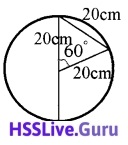Question 5.
If the arcs of the same lengths in the two circles subtend angles 65° and 110° at the centre, find the ratio of their radii.
We have l = rθ, the radius and angle are inversely proportional. Therefore;Question 6.
Find the values of the other five trigonometric functions in the following; (2 score each)

1. cos x = $$-\frac{3}{5}$$, x lies in the third quadrant.
2. cot x = $$-\frac{5}{12}$$, x lies in the second quadrant.
3. sin x = $$\frac{1}{4}$$, x lies in the second quadrant.

1. Given;
cos x = $$-\frac{3}{5}$$2. Given;
cot x = $$-\frac{5}{12}$$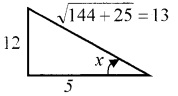3. Given;
sin x = $$\frac{1}{4}$$; cosecx = 4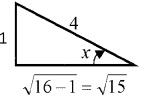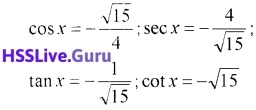Question 7.
Find the value of the trigonometric functions. (2 score each)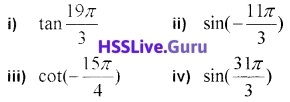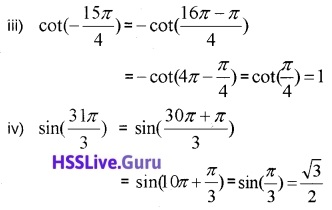Question 8.
Find the value of the following.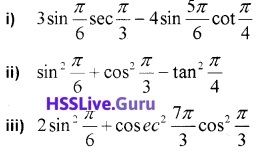iv) sin 75°
v) tan 15°iv) sin 75° = sin(45° + 35°)
= sin 45° cos30° + cos45° sin 30°v) tan 15° = tan(45° – 30°) = $$\frac{\tan 45^{\circ}-\tan 30^{\circ}}{1+\tan 45^{\circ} \tan 30^{\circ}}$$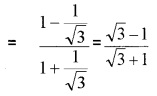Question 9.
Find the principal and general solution of the following.

1. sin x = $$\frac{\sqrt{3}}{2}$$
2. cosx = $$\frac{1}{2}$$
3. tan x = $$\sqrt{3}$$
4. cos ecx = -2

1. Given; sin x = $$\frac{\sqrt{3}}{2}$$ = sin $$\frac{\pi}{3}$$
General solution is; x = nπ + (-1)n$$\frac{\pi}{3}$$,
n ∈ Z
Put n = 0, 1 we get principal solution; x = $$\frac{\pi}{3} ; \frac{2 \pi}{3}$$.

2. Given; cosx = $$\frac{1}{2}$$ = cos $$\frac{\pi}{3}$$
General solution is; x = 2nπ ± $$\frac{\pi}{3}$$, n ∈ Z
Put n = 0, 1 we get principal solution;
n = 0 ⇒ x = $$\frac{\pi}{3}$$; n = 1 ⇒ x = 2π – $$\frac{\pi}{3}$$ = $$\frac{5\pi}{3}$$.

3. Given; tan x = $$\sqrt{3}$$ = tan$$\frac{\pi}{3}$$
General solution is; ⇒ x = nπ + $$\frac{\pi}{3}$$, n ∈ Z
Put n = 0, 1 we get principal solution;
n = 0 ⇒ x = $$\frac{\pi}{3}$$; n = 1 ⇒ x = π + $$\frac{\pi}{3}$$ = $$4\frac{\pi}{3}$$.

4. Given; cosecx = -2
⇒ sin x = $$-\frac{1}{2}$$ = – sin $$\frac{\pi}{6}$$ = sin(-$$\frac{\pi}{6}$$ )
General solution is; x = nπ – (-1)n $$\frac{\pi}{6}$$, n ∈ Z
Put n = 1, 2 we get principal solution;